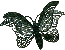Album of Algorithms and Techniques Recipe Index A All pairs number A.I+A.J=X APPROX_SUBSET_SUM - approximation algorithm,    subset-sum problem ATRIAN - area of triangle (stable method) B BINARY_SEARCH - search BITARRAY2D - bit array as decimal number BOYER_MOORE_MATCHER - string-matching C CABS - complex modulus CADD - complex addition CDIV - complex division CEILING -XCMUL - complex multiplication COMPLEX - creates a complex number COS - cosine function COUNTING_SORT - sorting Creating a function returning constant CSQRT - complex square root CSUB - complex subtraction D DDPOLY - evaluation polynomial & its derivatives DDPOLY1 - evaluation polynomial and its 1st     derivative DECOMPRESS DIOPHANT - diophantine linear equation Determinant of a matrix DPS - dynamic programming, subset-sum     problem D2BITARRAY - decimal number as bit array D2R - decimal to roman E E - Euler's number e ECONST - first 200 digits of e Euler's constant gamma EXACT_SUBSET_SUM - exact algorithm,    subset-sum problem EXEUCLID - extended form of Euclid's algorithm EXP - exponential function GENERAL_FIB - general formula for Fibonacci    numbers F FACT - factorial FADD - addition fractions FCOMP - comparison fractions FDIV - division fractions FIB - Fibonacci numbers FIND - selecting the Kth smallest FLOOR -XFMUL - multiplication fractions G GAMMA_CONST - first 60 digits of gamma GCD - Euclid's GCD algorithm GENSUB - generation of all subsets with K    elements Gray code GS - greedy algorithm, subset-sum problem H HEAPSORT - sortingHEAPSORT_RADIX3 - sorting HERON - area of triangle (unstable method) HORNER - evaluating polynomials I IMAGINARY - imaginary part of complex number INTERPOLATION_SEARCH - search Inverse of a matrix J K KMP_MATCHER - string-matching L LCG - linear congruential generator LCM - least common multiple LN - natural logarithm LN2 - first 200 digits of LN(2) LN2P - LN(2) LN10 - first 200 digits of LN(10) LOCATE - search an array of abscissas LUBKSB - routine for solution of linear    algebraic equations LUDCMP - LU decomposition M Matrix multiplication - definition Matrix multiplication - Winograd MINIMAX - min. and max. (recursive) MINIMAX - min. and max. (iterative) MINANDMAX - min. and max. (practical) MERGING MOD MODE - the most frequently occuring value MODIFIND - selecting the Kth smallest Modular linear equations N NAIVE_STRING_MATCHER - string-matching NCOMB - the number of combinations O P PERMUTE - generation of permutation sequences PI - number Pi PICONST - first 200 digits of Pi POLINT - polynomial inter/extra-polation POLYADD - polynomial addition POLDIV - polynomial division POWER - integer exponent PRIMES - the first K primes PRIMALITY_TEST - testing for primality Q QUICKSORT - sorting      QUICKSORT_ITERATIVE      QUICKSORT_RECURSIVE      QUICKSORT sophisticated      QUICKSORT - sorting in descending order R RATINT - rational function inter/extra-polation RCMUL - real multiplier of complex number REAL - real part of complex number REAL_STRING_MATCHER - string-matching REPOWER - power, real exponent R2D - roman to decimal S SAMPLING_B - random sampling, Bentley SAMPLING_K - random sampling, Knuth Search in associative arrays SELECT - selecting the Kth smallest SEQUENTIAL_SEARCH - search SEQUENTIAL_SEARCH_WITHOUT_SENTINEL SHELLSORT - sorting SHUFFLE - random permutation Shuffling array according to certain permutation SIN - sine function Solution of linear algebraic equations SPARSE_POLYADD - polynomial addition for    sparse polynomials SPLINE - computes the second derivatives    of the interpolating function SPLINT - spline interpolation function SQRT - square root of positive real number SQRT5 - first 2100 digits of SQRT(5) SQRTP2AQ2 - SQRT(P**2 + Q**2) STIRLING - Stirling's formula U W X Y Z